Bottom line: Learn how the SUBTOTAL function works in Excel to create formulas that calculate results on the visible cells of a filtered range or exclude hidden rows.

Skill level: Beginner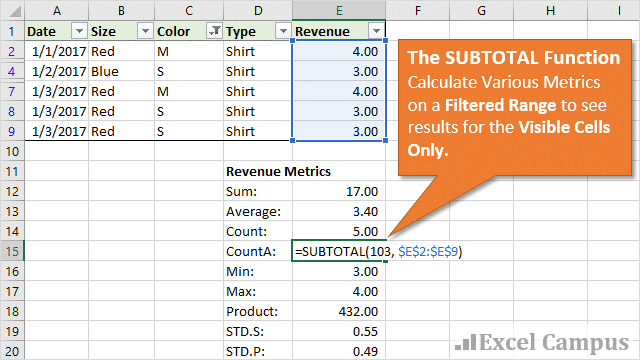## The SUBTOTAL Function Explained

The SUBTOTAL function is a very handy function that allows us to perform different calculations on a filtered range.  The most common use is probably to find the SUM of a column that has filters applied to it.

The SUBTOTAL function will display the result of the visible cells only.  This is great for tying out numbers in a filtered column to summary reports and pivot tables.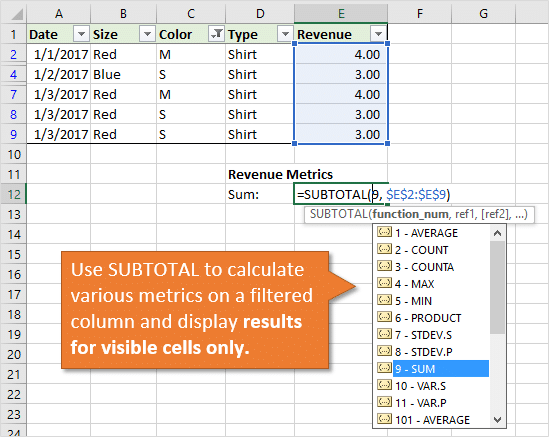SUBTOTAL actually gives us eleven (11) different functions to choose from.  We can use it to return the SUM of the filtered range, or any of the following calculations.

1. AVERAGE
2. COUNT
3. COUNTA
4. MAX
5. MIN
6. PRODUCT
7. STDEV.S
8. STDEV.P
9. SUM
10. VAR.S
11. VAR.P

That means we can use SUBTOTAL for any of the different calculation types listed above.  However, only ONE of the calculation types can be used in the formula to return the result to a single cell.

## How to Write a SUBTOTAL Formula

The SUBTOTAL function is pretty simple.  It just has two required arguments.

### Argument #1 – function_num

The first argument is the function number.  This allows us to specify which of the eleven calculation types we want to use, and we can select the number from the drop-down list in the formula editor.To select an item in the list, double-click it or press the Tab key.

Quick Tip: If the drop-down list disappears, you can press Alt+Down Arrow to view it again.

You probably noticed that the calculation types are listed twice.  One set that is single digit number, and another set that stars with 101.

• The 1-11 functions will include hidden rows
• The 101-111 functions will ignore hidden rows.

Hidden rows are different from filtered rows.  Both calculation types will excluded rows that are not visible due to filtering.  However, if we were to hide additional rows in a filtered range then the 1-11 functions would still include these hidden rows in the result.  The 101-111 functions would not include the hidden rows.

So, if you only want to see the results for visible rows, regardless of how the rows are hidden, then use the 101-111 functions.

The argument is specified by typing the single number 1-11, 101-111 in formula.  When we select an item from the drop-down list, it will automatically put the number in the formula.

We can also reference a cell that contains the number of the function.  This can be used for a dynamic formula where you might want the user to select the metric from a drop-down list.

### Argument #2 – ref1

The second argument is the range (column) of cells we want to perform the calculation on.  We can simple select the range of cells or type in the range reference.This range will typically be a single column that has filters applied to it.

Don't forget to make the column and/or row references absolute (F4 key), depending on if you are going to copy the formula down/across.

We can also add additional ranges to the formula.  We can include additional columns that are not contiguous (next to each other), by adding additional ref ranges to the formula.  These range references are separated by a comma.  We can add up to 254 additional range references.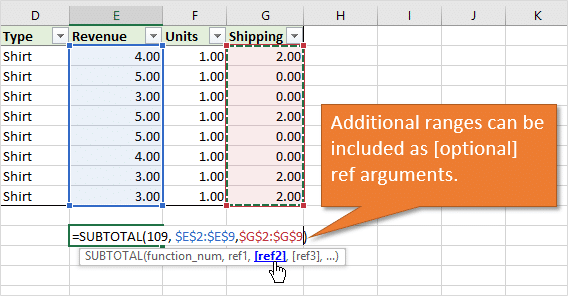## Apply Filters To See The Calculation on Visible Cells

Now that we have the SUBTOTAL function written, we can apply filters to multiple columns in the range.  The SUBTOTAL function will return the results of the visible cells only.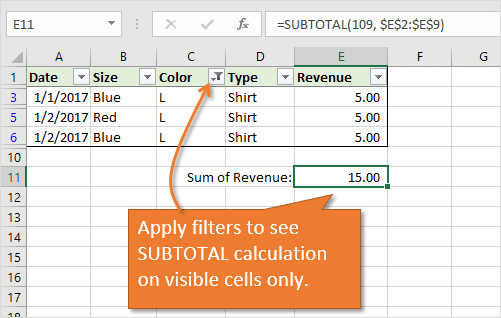Excel Tables also use the SUBTOTAL function in the Totals Row of a Table.  You can select one of the calculation types from a in-cell drop-down menu in the Totals Row, and the SUBTOTAL function will automatically be created for you.  This is one of the many time saving benefits of using Excel Tables.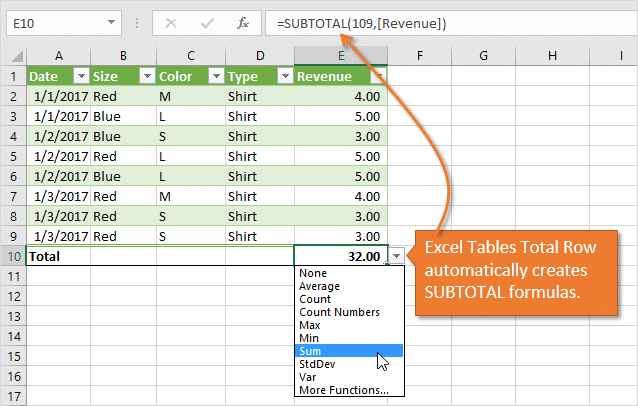Bonus tip: The SUBTOTAL function can also automatically be created with the AutoSum feature when the range we are applying it to is filtered.

## The SUBTOTAL Metrics Macro

Sometimes we want to use the SUBTOTAL function to calculate more than one calculation type on a column.  For example, we might want to see the Sum, Min, Max, Count, and Average of the column for quick analysis.

Typing a SUBTOTAL formula for each calculation type can take some time.  So, I wrote a macro that creates all the formulas for us with just a few clicks.

The SUBTOTAL Metrics Macro creates a summary table on the sheet with a SUBTOTAL formula for each calculation type based on range that you specify.  Here is a quick screencast so you can see it in action.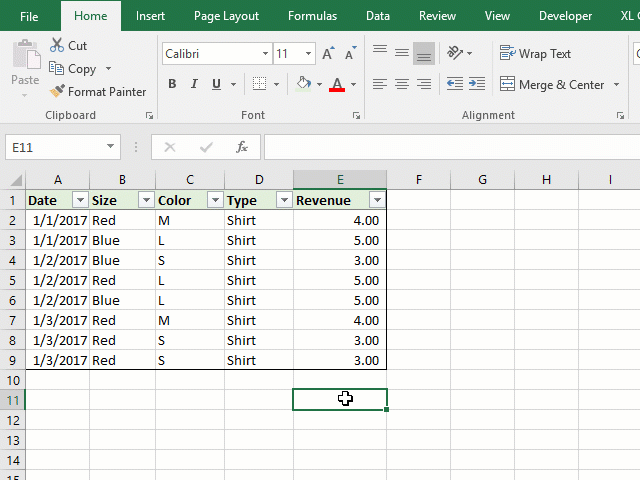I have a separate post that explains the macro in detail, including how to modify it for your needs.  You can also download the file that contains the macro there.

## What About the AGGREGATE Function?

There were a few comments posted below about the AGGREGATE function, so I'm adding a section here.

The AGGREGATE function was introduced in Excel 2010.  It works similar to the SUBTOTAL function, but has some additional features that allow us to ignore cells with errors values and other SUBTOTAL or AGGREGATE formulas in the ref range.  It's another great function to add to your tool box.

Even though AGGREGATE has more features, it's still good to know SUBTOTAL for a few reasons:

1. If you have any users still on Excel 2007, then the AGGREGATE function will not work for them.  You have to use SUBTOTAL.
2. SUBTOTAL is to AGGREGATE, as VLOOKUP is to INDEX/MATCH.  SUBTOTAL is like the baby brother of AGGREGATE.  Similar to the relationship between VLOOKUP and INDEX/MATCH.  My guess is that VLOOKUP is used over 95% of the time by users, even though INDEX/MATCH offers a lot of addtional benefits (checkout my free videos series on the lookup formulas to learn more about the differences).  The same is probably true with SUBTOTAL, especially since Excel uses the formula in a lot of different Excel features.
3. Excel automatically generates the formulas, as I mentioned above, so it is very likely you will see this formula in an Excel file.  Excel creates formulas with the SUBTOTAL function in the following Excel features/commands:
1. The Subtotal command (Data tab > Subtotal).
2. The AutoSum command on a filtered range (Home tab > AutoSum or Alt+=)
3. The Totals Row of a Table (Ctrl+Shift+T).

Excel likes to create these formulas for us, so it's good that we know they work. 🙂

With all that said, AGGREGATE is still AWESOME, and one every Excel user should know.  I will do a separate post that explains the differences in more detail.

## SUBTOTAL Versatility

The SUBTOTAL function is a great one to have in your Excel tool belt, especially when working with filtered ranges of data.

Do you have any tips or tricks for using the SUBTOTAL function?  Please leave a comment below with questions or suggestions.  Thank you!

•Bipin Damania says:

How can I use subtotal with sumif or vis-a-versa ?

•Kim says:

My formula is
=COUNTIF(F6:F16,”*YES*”)/(COUNTA(F6:F16))
how can I adjust this to show only the percentage of YES of my filtered rows?

•Elise says:

How can I lock a subtotal value after I’ve removed a filter? Ie – I’ve subtotal formula on range of filtered cells and got the value I needed. Then I want to filter a different data set and compare the filtered subtotals without writing each subtotaled value down. Please help!

•Andrew says:

All the examples sum a column of numbers. I tried to do the same for a row of numbers, but it didn’t work. Is there something I am missing?

January February March April May 2018 YTD
3,525 3,122 4,082 3,814 3,965 45,832

In the example above the months Jun to Dec are hidden, so I expected the subtotal function to exclude them, but it didn’t. The columns were hidden with right-click / hide.

Here is the formula I am using: =SUBTOTAL(109, K10:V10)

•Bipin Damania says:

How can I use subtotal with sumif or vis-a-versa

•Dave says:

Hey there!!

Is there any way I can embed other functions within SUBTOTAL?

I’m trying to count unique instances of a value in a filtered list. I came up with:

=COUNT(IF(FREQUENCY(range,range)>0,1))
or
=SUMPRODUCT(1/COUNTIF(data,data))

…which seem to work fine, but as I need it for a filtered list, how can I use these to count only the filtered data?

Thanks!!!

•Dawna says:

Hi Jon,

I’ve created a pivot table to show YTD revenue vs labor by month (so right now it reports Jan-Jul 2018, one column each for revenue and labor) with a row for YTD grand total at bottom. Below that I want to report YTD monthly avg. I’ve been able to get the results I need two ways so far, but each way requires either unhiding rows when I refresh for the next month OR manually adjusting the avg formula to divide by current # of months.

Method 1: Create appropriate pivot table but remove Grand Total field and leave enough blank rows to accommodate future months (Aug-Dec); hide rows for future months; add manual row for YTD Grand Total using =Subtotal(9,…) and another row below for YTD Mo Avg using =Subtotal(1,…). Unhide row for next month when it is time to refresh data.

Method 2: Create appropriate pivot table but keep default Grand Total field; add rows needed for future months below Grand Total (in this case 5 for Aug-Dec) and then hide. Create row to calculate Mo Avg using structured reference divided by current number of months (7). Refresh table after adding August data, which will automatically expand one row, then edit Avg formula to divide by 8.

Is there a better option that will eliminate any manual tinkering?

Thanks,
Dawna

•JOHN says:

Is there a way to ignore a filter and still show the sum? I would like to filter by date for ease of entering more data, but i want to Sum all the rows, not just the visible rows.

•Diana says:

HI! I’ve searched and searched, and can’t find a formula to count the number of rows in a filtered list containing TODAYS DATE or GREATER THAN TODAY’S DATE. Any help would be very much appreciated!!! THanks!

•Jon Acampora says:

Hi Diana,

You can use the COUNTIF function for this and combine the criteria with a comparison operator. Here is an example.

`=COUNTIFS(A2:A100,">="&TODAY())`

The TODAY function returns today’s date. I’ll write up a post on this.

•Jon Acampora says:

Hi Diana,
Sorry, I didn’t fully read your question. For a filtered range you can use the technique described on the following page.

https://exceljet.net/formula/count-visible-rows-only-with-criteria

You will just change the comparison operator in the criteria section to >=

I hope that helps. 🙂

•Rick says:

Is there a way to use INDEX instead of OFFSET inside SUBTOTAL to return a Boolean array indicating whether or not a row is visible due to a filter?

For Example,

returns such an array for a table of data.

However,

=SUBTOTAL(103,INDEX(tblTest[ID],N(IF({1},ROW(INDIRECT(“1:”&ROWS(tblTest)))))))

returns an array of #VALUE!. When evaluating the results of INDEX() I’m getting an array of the data from tblTest[ID] but it seems SUBTOTAL is interpreting these as numbers and not references.

•Lschwa01 says:

Please add a section on copying the results. When I use the 2 option on the processed data I get the summary result lines. There’s a way to copy only those lines but I can’t remember it. Thanks for all of your tips.

•shiva says:

Hi John,

Thanks for explaining the difference between 1-11 & 101 to 111.
When i tried to use the SUM formula using single digit it actually works on including hidden rows to the total only when the range is not filtered. If we apply any filters to the range it is considering only visible cells but not hidden rows.
Am i doing any mistake here.
Because normal sum formula would serve the purpose of including hidden rows.

Shiva

•Carrie says:

Thanks for explaining the difference between 1-11 vs 101-111 function. I never could figure out the difference since I usually use the Alt + = on a table. Cheers~!

•Jon Acampora says:

Thanks Carrie! Interesting enough, Excel uses the 109 function when you use AutoSum at the bottom of an Excel Table. If you use AutoSum at the bottom of a regular range that has filters applied, Excel uses function 9. Hmm… 🙂

•THOMAS K. says:

Hi Jon

I have around 20 columns and valid 5000 rows in an excel sheet with filter enabled for each column.

The first column ‘A’ is for entering the date (from 01 Jan to 31 Dec).
For other columns I have a lot of criteria’s to add and filter if required.

My subject is, in all rows of a specific column (let’s say column K) there will be several “YES”, “NO” or some digits “10”, “20” which is a result for certain tasks. I want to calculate and show the percentage of these results “YES”, “NO”, “10” or “20” in a specific cell in the title row. ie. If there is 50 nos of “YES” and 25 nos of “NO” and 25 nos of “10”, I want to display “YES”=50%, “NO”= 25% & “10”=25%. The important thing here is that, this percentage shown should not be the total calculated from the 5000 rows, BUT ONLY WHAT IS SHOWING FILTERED AS PER THE DATE’S COLUMN ‘A’. (means, the percentage shown should be as per the view mode)

Can you help me?

•Kirk says:

Great information, Jon!

I have found that AGGREGATE function suits my needs better when I’m using large tables of data.

I’d like to hear your thoughts on SUBTOTAL vs. AGGREGATE.

•Jon Acampora says:

Hi Kirk,
Yes, AGGREGATE is another great function to know. I added a section above that explains some of the differences, and why it’s still good to know SUBTOTAL. I will write a separate post on AGGREGATE. Thank you!

•David says:

Jon,

Great tip.
Thanks for sharing the tip!

•Jon Acampora says:

Thanks David! 🙂

•Juan says:

Thank you very much John for sharing this tip, it’s a pleasure to read such a great-explained trick!

•Jon Acampora says:

Thank you Jaun! 🙂

•MF says:

Nice blog post! Very detail in explaining the SUBTOTAL.

SUBTOTAL 103 can be used for creating a sequential number for visible rows only. Here’s the blog post:
https://wmfexcel.com/2015/05/09/sequential-number-for-visible-rows-only/

Hope you like it. 🙂

•Jon Acampora says:

Thanks for sharing MF. Great trick! 🙂

•Matt says:

Why not =AGGREGATE()? What are the differences besides AGGREGATE having more options for ignoring cells.

•Jon Acampora says:

Hey Matt, Great question! I added a section above that explains a few of the differences between SUBTOTAL and AGGREGATE. I also explain why it’s good to still know SUBTOTAL, even though AGGREGATE has more features. I will write a separate post that details the differences. Thanks!

Generic filters
Exact matches only
Filter by Custom Post TypeLearn 10 great Excel techniques that will wow your boss and make your co-workers say, "how did you do that??"